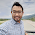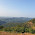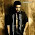# With 100% modulation,ratio of side band power to total power transmitted in an amplitude modulated wave is

B) 1/3

C) 1/2

D) 1/4

Note: Post your answers with Option name and reason of your answer so that others can able to understand and if you want to receive answers from other candidates, click notify me below the comment form.

View Answers                                       Post your Answer

#### 4 comments :

1.2.ratio of sideband power to total power= u^2/(2+u^2) where u=modulation index. Since u=1, so ratio=1/3. ans is b

3.4.here as we know total power pt = pc (carrier power )+ pc.m^2/2 ( sideband or modulating power ) . now at m = 1 we can calculate modulating power/total power